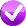# Building Java Programs, 3rd edition

## Lab: Random Numbers

Except where otherwise noted, the contents of this document are Copyright 2013 Stuart Reges and Marty Stepp.

lab document created by Marty Stepp, Stuart Reges and Whitaker Brand

# Lab goals

Goals for this problem set:

• use `Random` objects to produce random numbers
• Where you see this icon, you can click it to check the problem in Practice-It!# `Random` methods

To use these methods, you need a variable of type `Random` in scope:

```Random randy = new Random();
int aRandomNumber = randy.nextInt(10);  // 0-9
```
Method name Returns...
`nextInt()` a random integer
`nextInt(max)` a random integer between 0 (inclusive) and max (exclusive)
`nextDouble()` a random real number between 0.0 and 1.0
`nextBoolean()` a random `boolean` value: `true` or `false`

# Exercise : Random expressions

Fill in the boxes to produce expressions that will generate random numbers in the provided ranges. Assume that the following `Random` variable has been declared:

```Random rand = new Random();
```
 Example: a random integer from 1 to 5 inclusive: ```rand.nextInt(5) + 1 ``` a random integer from 0 to 3 inclusive: `rand.nextInt(4)` a random integer from 5 to 10 inclusive: ```rand.nextInt(6) + 5 ``` a random integer from -4 to 4 inclusive: ```rand.nextInt(9) - 4 ``` a random even integer from 16 to 28 inclusive: (Hint: To get only even numbers, scale up.) ```rand.nextInt(7) * 2  + 16 ```

# Exercise : makeGuessesWrite a method named `makeGuesses` that will output random numbers between 1 and 50 inclusive until it outputs one of at least 48. Output each guess and the total number of guesses made. Below is a sample execution:

```guess = 43
guess = 47
guess = 45
guess = 27
guess = 49
total guesses = 5
```

Try solving this problem in Practice-It! from the link above.